# Study Guide

## Field 047: Science—Physics Sample Multiple-Choice Questions

The following reference material will be available to you during the test:

##### Objective 0001 The Nature and Processes of Science (Standard 1)

1. A scientist makes a claim about the characteristics of the energy levels of the electrons in a metal. The scientist then makes a prediction about the metal by arguing that if the claim is true, the metal should display specific measurable electric properties when placed in an oscillating magnetic field. Which of the following best characterizes the process that was used to go from making the claim to making the prediction?

1. revising a theory
2. using deductive reasoning
3. refining results
4. changing an experimental design
Correct Response: B.
This question requires the examinee to demonstrate knowledge of the characteristics, assumptions, and goals of science. Deductive reasoning is the process of reasoning from one or more statements to reach a logically certain conclusion. One of the characteristics of science is that scientists use deductive reasoning to start from general statements about the natural world and infer the results of specific experiments assuming those statements are true. Deductive reasoning is the process being used to go from the claim to the prediction in this question.

##### Objective 0002 Central Concepts and Connections in Science (Standard 2)

2. The three examples in the table below best illustrate which of the following concepts common to science and engineering?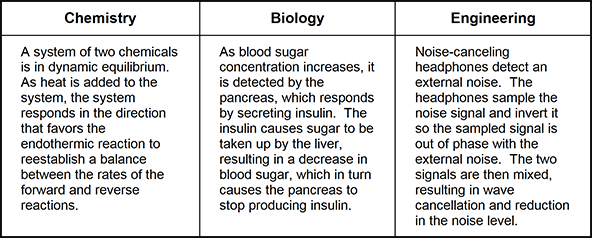1. structure and function
2. negative feedback
3. scale and quantity
4. exponential decay
Correct Response: B.
This question requires the examinee to demonstrate knowledge of the unifying concepts and processes that cut across the sciences and engineering. Feedback is a common concept in many scientific and engineering systems. A feedback loop monitors a system's output and uses the result to adjust the input. The feedback is negative if it tends to slow down or reduce the magnitude of the output. This is the case in each of the three examples given.

##### Objective 0003 Motion and Forces (Standard 3)

3. Use the graph below to answer the question that follows.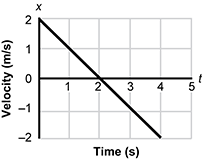The graph shows the velocity of a particle moving in one dimension with respect to time. The particle is at the origin at t = 0 s. Which of the following equations gives the position of the particle with respect to time?

1. x = –0.5t2 + 2t
2. x = –2t2 + 2t
3. x = t2 – 2t
4. x = 2t2+ 2t
Correct Response: A.
This question requires the examinee to demonstrate knowledge of the representation of motion using graphs, algebra, and calculus. Acceleration is the rate of change of velocity with respect to time, or the slope of the velocity with respect to time graph. For this graph the acceleration is a constant a = – 1 m/s2. The initial velocity from the graph is vi = 2 m/s. The problem states that the initial position of the particle is xi = 0 m. Putting these values into the formula for the position with respect to time for a particle undergoing constant acceleration gives x = –0.5t2 + 2t.

##### Objective 0003 Motion and Forces (Standard 3)

4. Use the diagram below to answer the question that follows.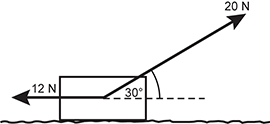A rope with a tension of 20 N pulls on a 4 kg box across a rough surface. The frictional force on the box is 12 N. Which of the following is closest to the coefficient of friction between the box and the surface?

1. 0.2
2. 0.3
3. 0.4
4. 0.6
Correct Response: C.
This question requires the examinee to demonstrate knowledge of characteristics of the gravitational force and frictional forces. The coefficient of friction is the ratio of the magnitude of the frictional force to the magnitude of the normal force on the block, or. The magnitude of the normal force can be obtained by noting that the normal force pushing the box up plus the component of the tension in the rope pulling the box up must balance the gravitational force pulling the box down. This gives the magnitude of the normal force equal to (4 kg) (9.80 m/s2) – (20 N) sin 30° = 29 N. Then the coefficient of friction is (12 N) / (29 N) = 0.41 = 0.4

##### Objective 0003 Motion and Forces (Standard 3)

5. Use the diagram below to answer the question that follows.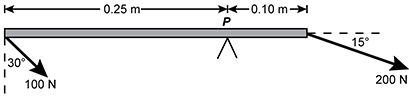The diagram shows the two forces acting on a rigid bar free to pivot about point P. If torques in the clockwise direction are negative, what is the net torque about the pivot point, P?

1. 25 cos 30° – 20 sin 15°
2. –25 cos 30° – 20 sin 15°
3. 25 sin 30° – 20 cos 15°
4. –25 sin 30° – 20 cos 15°
Correct Response: A.
This question requires the examinee to demonstrate knowledge of torque and its application to static systems. One way to calculate the torque due to a force at a point is to find the product of the distance from the point and the component of the force perpendicular to the line where force is applied. The sign of the torque is determined by which way the force tends to rotate the object. For the force at the left of the diagram, this results in a torque of + (0.25 m) (100 N) cos 30° = + 25 N·m cos 30°; positive because this force tends to rotate the bar counter-clockwise. For the force at the right, we get a torque of – (0.10 m) (200 N) sin 15° = – 20 N·m sin 15°; negative because this force tends to rotate the bar clockwise. Adding the two torques together gives the net torque about point P.

##### Objective 0004 Energy and Momentum (Standard 4)

6. Use the graph below to answer the question that follows.A child has a small bow and arrow set. The graph shows the force required to pull the string on the bow a given distance. If the child places the end of an arrow of mass 0.05 kg on the string and pulls the string on the bow a distance of 0.3 m and releases, what speed will the arrow have when it leaves the string?

1. 6.0 m/s
2. 9.0 m/s
3. 13.4 m/s
4. 19.0 m/s
Correct Response: C.
This question requires the examinee to demonstrate knowledge of conservation of energy and the work-energy theorem. The magnitude of work supplied by the child in drawing the bow the given distance is equal to the area under the force versus distance graph, which is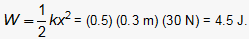This energy then becomes the kinetic energy of the arrow,, when the arrow is released. Solving for the speed of the arrow, v, gives 13.4 m/s.

##### Objective 0004 Energy and Momentum (Standard 4)

7. A potter's wheel with a moment of inertia of 7.0 kg·m2 is spinning freely at 22 rpm. The potter drops a 3.0 kg lump of clay on to the wheel, where it sticks a distance of 0.50 m from the axis of rotation. What is the subsequent angular speed of the wheel?

1. 9.3 rpm
2. 13 rpm
3. 18 rpm
4. 20 rpm
Correct Response: D.
This question requires the examinee to demonstrate knowledge of the conservation of angular momentum. The angular momentum of the system is conserved; Iiωi = Ifωf, where I is the moment of inertia and ω is the angular speed. The final moment of inertia is the initial moment of inertia of the wheel plus mr2 for the lump of clay; If = (7.0 kg·m2) + (3.0 kg) (0.50 m)2 = 7.75 kg·m2. Solving the conservation of angular momentum equation for the final angular speed gives ωf = (7.0 kg·m2) (22 rpm) / (7.75 kg·m2) = 20 rpm.

##### Objective 0005 Electricity and Magnetism (Standard 6)

8. Use the diagram below to answer the question that follows.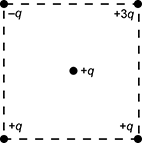Charges are located on each corner of a square and a fifth charge is in the center of the square, as shown in the diagram. In what direction is the net force on the charge located in the center of the square?

1. toward the top of the square
2. toward the bottom of the square
3. toward the right of the square
4. toward the left of the square
Correct Response: D.
This question requires the examinee to demonstrate knowledge of electric charge, electrostatics, the electric force, and Coulomb's law. Coulomb’s law shows that the magnitude of the electric force is proportional to the magnitude of each of the charges. Let F represent the size of the force from a single charge located on a vertex of the square on the charge located in the center of the square. Like charges repel and unlike charges attract. Then the +3q charge exerts a force of size 3F towards the bottom left corner. The +q charge at the lower left corner exerts a force F toward the upper right corner of the square. These forces act along the same line. When added, they result in a force of magnitude 2F towards the bottom left corner. The +q charge at the lower right corner exerts a force F toward the top left corner. The –q charge at the upper left corner also exerts a force F towards the top left corner. These forces add to make a force of magnitude 2F towards the top left corner. Due to the symmetry of the square, the forces add to produce a force which is purely toward the left of the square, since the vertical components cancel and the horizontal components add.

##### Objective 0005 Electricity and Magnetism (Standard 6)

9. Use the diagram below to answer the question that follows.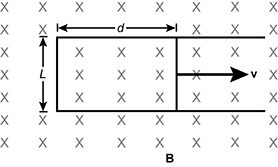A metal rod is forced to move at a constant speed, v = 0.62 m/s, to the right along two parallel metal rails connected by a strip of metal at their left ends, as shown. A magnetic field of magnitude B = 430 mT is directed into the plane of the diagram as indicated by the x-marks. The rails are separated by L = 0.71 m and the rod is located at d = 0.83 m from the left ends of the rails at a particular point in time. What electromotive force is generated at this time?

1. 0.16 V
2. 0.19 V
3. 0.22 V
4. 0.25 V
Correct Response: B.
This question requires the examinee to demonstrate knowledge of Faraday's law of induction and induced electric fields and electromotive force. Faraday's law says that the size of the electromotive force is equal to the rate of change of the magnetic flux through the loop. The magnetic flux in this case is just the size of the magnetic field times the area of the loop. The flux is changing because the area of the loop is changing. The flux at any time t is given by BLvt. Differentiating this formula gives the rate at which the flux changes, so the size of the electromotive force is BLv = (0.43 T) (0.71 m) (0.62 m/s) = 0.19 V.

##### Objective 0006 Vibrations and Waves (Standard 7)

10. Use the diagram below to answer the question that follows.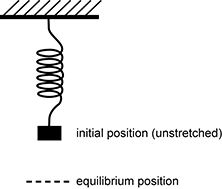A spring with a spring constant of 100 N/m is attached to the ceiling and hangs vertically. An object of mass 2.0 kg is attached to the end of the spring and held without stretching the spring. The object is then released from rest. How long will it take for the object to get back to its initial position?

1. 0.13 s
2. 0.89 s
3. 1.4 s
4. 7.1 s
Correct Response: B.
This question requires the examinee to demonstrate knowledge of the application of force and energy principles to simple harmonic motion and oscillating systems. Applying Newton’s 2nd law to the system shows that the mass will execute simple harmonic motion around the equilibrium position. The mass will return to the initial position after one full period of oscillation. Using the formula for the period of a mass on the end of a spring undergoing simple harmonic motion and substituting the given values results in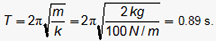##### Objective 0006 Vibrations and Waves (Standard 7)

11. A sound wave has a frequency of 680 Hz. If the speed of sound in air is 340 m/s, what is the distance between a region of maximum pressure (compression) and minimum pressure (rarefaction) of the sound wave in air?

1. 0.25 m
2. 0.5 m
3. 1 m
4. 2 m
Correct Response: A.
This question requires the examinee to demonstrate knowledge of the properties of sound waves. Using the relationship among the speed of a sinusoidal wave, its frequency and wavelength, v = f λ, we can calculate the wavelength for this sound wave: λ = v / f = (340 m/s) / (680 Hz) = 0.50 m. But a wavelength is the distance from a crest to the next crest on the wave. For a sound wave, this is from one compression to the next adjacent compression. The question asks for the distance from a crest (compression) to a trough (rarefaction) which is half a wavelength, or 0.25 m.

##### Objective 0007 Thermodynamics and Kinetic Theory (Standard 5)

12. Use the graph below to answer the question that follows.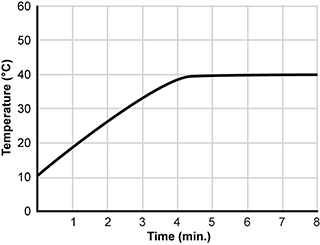An 80 g mass at an initial temperature of 100°C is placed in a thermally insulated container filled with 40 g of water. The temperature of the water with respect to time is shown. Which of the following expressions is equal to the specific heat of the mass [Cwater = 4.2 J/(g°C)]?

1. 1.1 J/(g°C)
2. 1.4 J/(g°C)
3. 2.1 J/(g°C)
4. 3.5 J/(g°C)
Correct Response: A.
This question requires the examinee to demonstrate knowledge of heat and temperature, specific heat, and methods
of heat transfer. The graph shows that the temperature of the object went from 100 °C to 40°C, resulting in an energy
change ΔQο = cmΔT = c(80 g)(60°C), where c is the object’s specific heat. Because the object and water are
in a thermally insulated container, this energy change must equal the energy change of the water, which is
ΔQw = (4.2 J/(g°C)) (40 g) (30°C). Equating these two expressions and solving for the specific heat of the object yields
c = 1.1 J/(g°C).

##### Objective 0007 Thermodynamics and Kinetic Theory (Standard 5)

13. An ideal gas enclosed in a container with a moveable piston undergoes an adiabatic expansion. Which of the following must occur as the gas expands?

1. The gas does work and its internal energy remains constant.
2. Thermal energy is added to the gas and its temperature remains constant.
3. The gas does work and its temperature decreases.
4. Thermal energy is added to the gas and its internal energy increases.
Correct Response: C.
This question requires the examinee to demonstrate knowledge of the mechanical equivalence of heat, thermodynamic work, and the first law of thermodynamics. During an adiabatic expansion of a gas, ΔQ = 0. As the gas expands, the pressure of the gas pushes on the piston in the same direction that the piston moves which shows that the gas does positive work. Using the first law of thermodynamics, ΔEint = ΔQ – ΔW, with ΔQ = 0 and ΔW positive, shows that ΔEint is negative or that the internal energy of the gas decreases during the expansion. For an ideal gas, the internal energy is proportional to the temperature, so the temperature of the gas decreases during the expansion.

##### Objective 0008 Modern Physics (Standard 8)

14. A handheld laser pointer has power P and emits light of frequency f. Which of the following equations represents r, the rate with respect to time of photons emitted by the laser in terms of Planck's constant, h?

1.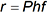2.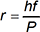3.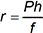4.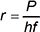Correct Response: D.
This question requires the examinee to demonstrate knowledge of energy of light quanta and the photoelectric effect. Power, P, is energy per unit time, and E = hf gives the energy for one photon. Dividing the energy per unit time by energy per photon gives the number of photons per unit time, or the rate of photons emitted by the laser which is.

##### Objective 0009 Science Instruction and Assessment (Standard 9)

15. During a unit on projectile motion, a student insists that the net force on a projectile is zero at the top of the projectile's trajectory. Which of the following strategies is likely to be most appropriate for the teacher to use to help address this misconception?

1. asking the student to multiply the mass of the object by its acceleration
2. having the student refer to the equation that describes the projectile's motion
3. asking the student to explain how gravity acts on the projectile along its trajectory
4. having the student determine change in potential energy along the trajectory